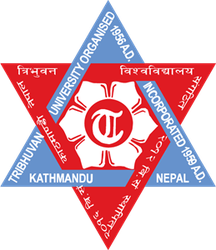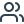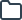Physics at Tribhuvan University Kathmandu | Flashcards & Summaries

### Select your language

Suggested languages for you:# Lernmaterialien für Physics an der Tribhuvan University Kathmandu

Greife auf kostenlose Karteikarten, Zusammenfassungen, Übungsaufgaben und Altklausuren für deinen Physics Kurs an der Tribhuvan University Kathmandu zu.

TESTE DEIN WISSEN

Is a vector necessarily changed if it is rotated through

an angle ?

Lösung anzeigen
TESTE DEIN WISSEN

Yes. A vector is defined by its magnitude and direction, so a vector can be changed by changing its magnitude and direction. If we rotate it through an angle, its direction changes and we can say that the vector has changed.

Lösung ausblenden
TESTE DEIN WISSEN

The minimum Number of co-linear vectors giving zero resultant is 2.  What about a minimum number of coplanar vectors giving zero resusltant.

Lösung anzeigen
TESTE DEIN WISSEN

3

Lösung ausblenden
TESTE DEIN WISSEN

Which of the following two statements is more appropriate:

Lösung anzeigen
TESTE DEIN WISSEN

Two velocities are added using the triangle rule because velocity is a vector quantity.

Lösung ausblenden
TESTE DEIN WISSEN

Can you add three unit vectors to get a unit vector ?

Does your answer change if two unit vectors are along

the coordinate axes ?

Lösung anzeigen
TESTE DEIN WISSEN

yes, taking three unit vectors as i , -i and j it sums up to be j which is a unit vector.

No my answer won't change.

Lösung ausblenden
TESTE DEIN WISSEN

Can you add two vectors representing physical

quantities having different dimensions ? Can you

multiply two vectors representing physical quantities

having different dimensions ?

Lösung anzeigen
TESTE DEIN WISSEN

Addition is not possible.

Multiplication is possible.

Lösung ausblenden
TESTE DEIN WISSEN

Can a vector have zero component along a line and still

have nonzero magnitude ?

Lösung anzeigen
TESTE DEIN WISSEN

Yes.

For example = 2i +0j

has magnitude of 2 along x direction.

Lösung ausblenden
TESTE DEIN WISSEN

The dot product of a vector A with the zero vector is

Lösung anzeigen
TESTE DEIN WISSEN

0

Lösung ausblenden
TESTE DEIN WISSEN

Two nonzero vectors are perpendicular, or orthogonal, if and only if their dot product is

Lösung anzeigen
TESTE DEIN WISSEN

0

Lösung ausblenden
TESTE DEIN WISSEN

Let A→=3i→+4j→. Write four vector B→ such that A→≠B→ but A=B

Lösung anzeigen
TESTE DEIN WISSEN

so the four different vectors can be

vec(B) = 3i-4j , -3i+4j, -3i-4j , 5k

Lösung ausblenden
TESTE DEIN WISSEN

In which case vector product and cross product will be equal?

Lösung anzeigen
TESTE DEIN WISSEN

All

Lösung ausblenden
TESTE DEIN WISSEN

A vector A points vertically upward to the plane and B points towards north. The vector product A

× B is

Lösung anzeigen
TESTE DEIN WISSEN

Along west

Lösung ausblenden
TESTE DEIN WISSEN

Can we have physical quantities having magnitude and

direction which are not vectors ?

Lösung anzeigen
TESTE DEIN WISSEN

It also should follow vector law of addition

No. A physical quantity having both magnitude and direction need not be considered a vector. For example, despite having magnitude and direction, current is a scalar quantity.

Lösung ausblenden•503 Karteikarten
•240 Studierende
•0 Lernmaterialien

## Beispielhafte Karteikarten für deinen Physics Kurs an der Tribhuvan University Kathmandu - von Kommilitonen auf StudySmarter erstellt!

Q:

Is a vector necessarily changed if it is rotated through

an angle ?

A:

Yes. A vector is defined by its magnitude and direction, so a vector can be changed by changing its magnitude and direction. If we rotate it through an angle, its direction changes and we can say that the vector has changed.

Q:

The minimum Number of co-linear vectors giving zero resultant is 2.  What about a minimum number of coplanar vectors giving zero resusltant.

A:

3

Q:

Which of the following two statements is more appropriate:

A:

Two velocities are added using the triangle rule because velocity is a vector quantity.

Q:

Can you add three unit vectors to get a unit vector ?

Does your answer change if two unit vectors are along

the coordinate axes ?

A:

yes, taking three unit vectors as i , -i and j it sums up to be j which is a unit vector.

No my answer won't change.

Q:

Can you add two vectors representing physical

quantities having different dimensions ? Can you

multiply two vectors representing physical quantities

having different dimensions ?

A:

Addition is not possible.

Multiplication is possible.

Q:

Can a vector have zero component along a line and still

have nonzero magnitude ?

A:

Yes.

For example = 2i +0j

has magnitude of 2 along x direction.

Q:

The dot product of a vector A with the zero vector is

A:

0

Q:

Two nonzero vectors are perpendicular, or orthogonal, if and only if their dot product is

A:

0

Q:

Let A→=3i→+4j→. Write four vector B→ such that A→≠B→ but A=B

A:

so the four different vectors can be

vec(B) = 3i-4j , -3i+4j, -3i-4j , 5k

Q:

In which case vector product and cross product will be equal?

A:

All

Q:

A vector A points vertically upward to the plane and B points towards north. The vector product A

× B is

A:

Along west

Q:

Can we have physical quantities having magnitude and

direction which are not vectors ?

A:

It also should follow vector law of addition

No. A physical quantity having both magnitude and direction need not be considered a vector. For example, despite having magnitude and direction, current is a scalar quantity.### Erstelle und finde Lernmaterialien auf StudySmarter.

Greife kostenlos auf tausende geteilte Karteikarten, Zusammenfassungen, Altklausuren und mehr zu.

## Das sind die beliebtesten Physics Kurse im gesamten StudySmarter Universum

##### physics

Ahfad University for Women

##### physics

British College of Osteopathic Medicine

##### Physics 2

Louisiana State University

##### Physics 1

Louisiana State University

##### physics

Arabian Gulf University

## Die all-in-one Lernapp für Studierende

##### Greife auf Millionen geteilter Lernmaterialien der StudySmarter Community zu##### Erstelle Karteikarten und Zusammenfassungen mit den StudySmarter Tools